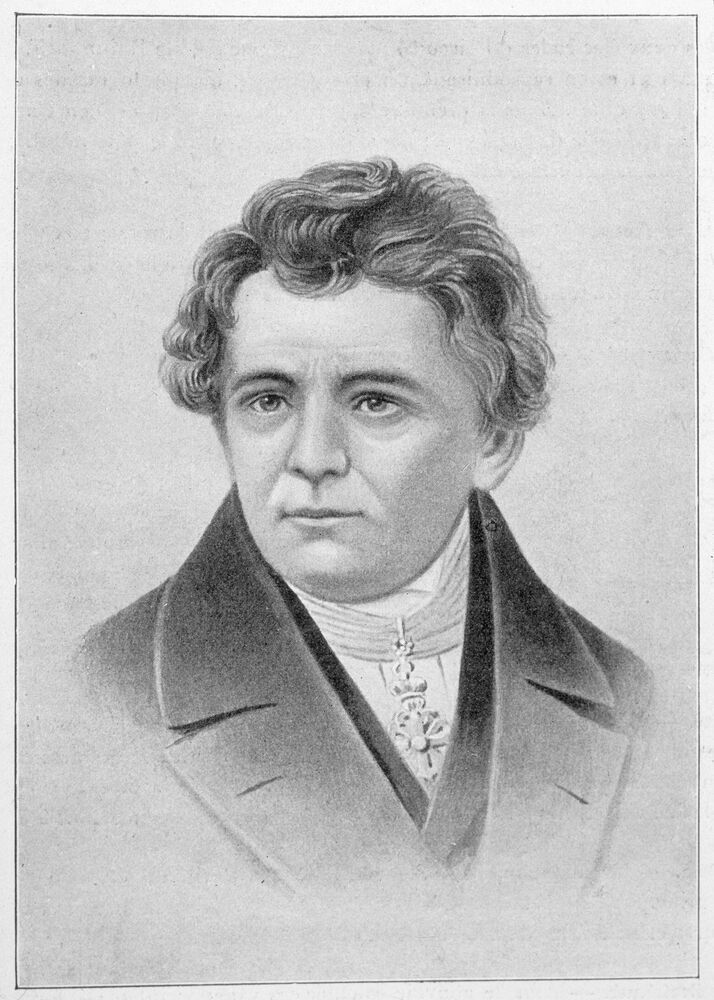## BASIC KNOWLEDGE - Ohm's lawThe meaning of Ohm’s law: Fundamentals at a glance

From Luke James Reading Time: 4 min |

Related Vendors

Ohm’s law is arguably one of the most important principles of power electronics and forms the basis of electronic circuit design. But what exactly is Ohm's law and what does the formula look like? Read this article to get Ohm's law explained.

### What is Ohm’s law?

Ohm’s law is a formula that’s used to calculate the relationship between voltage, current and resistance in an electrical circuit. These are three of the most critical circuit quantities in electronics and as such, Ohm’s law is an important principle that any aspiring electrical engineer must understand.

Today, Ohm's law is used within all branches of electrical engineering, especially within electronic circuit design. It’s used to calculate the value of resistors required in circuits, and it can also be used for determining the current flowing in a circuit where the voltage can be measured easily across a known resistor.

Ohm’s law is also used in an abundance of calculations in all forms of electronic circuit design, from the most basic to the highly complex. In fact, anywhere current flows, Ohm’s law applies.

### Ohm's law definition

A law stating that electric current is proportional to voltage and inversely proportional to resistance.

### What is the formula for Ohm’s law?

Ohm's law states that the current through a component, such as a resistor or a diode, is equal to the voltage across that component divided by that compound’s resistance. This can be expressed in three simple formulas that can be used interchangeably (see Figure 1)

Each of these formulas is essentially the same. Neither one of them is recognized as the “official” one; all three are used by various sources.

The interchangeability of the equation also means that it is sometimes represented as a triangle, where V (voltage) is placed on the top section, I (current) is placed bottom left, and R (resistance) is placed bottom right.

Watch this video to see Ohm's law explained with a practical example of how to use it in a simple circuit, in a series circuit and also in a parallel circuit:

### The history of Ohm’s law

In 1826, Georg Simon Ohm published papers giving a mathematical model for the way the circuits conducted heat. In May 1827, Ohm published Die galvanische Kette, mathematisch bearbeitet, which described the relationship between electromotive force, current, and resistance later known as Ohm's law. This work drew considerable inspiration from Jean-Baptiste Joseph Fourier’s work on heat conduction.Ohm’s law was defined by Georg Simon Ohm (1789 - 1854), a German physicist and mathematician. (Source: Archivist - stock.adobe.com)

Ohm used a galvanometer to measure current and knew that the voltage between the thermocouple terminals was proportional to the junction temperature. He then added test wires, diameter, and material to complete the circuit and found that his data could be modeled through the Ohm’s law equation.

Despite critics reacting to his work with hostility, describing it as fantasy, Ohm's law is now recognized as one of the most important of the early quantitative descriptions of the physics of electricity. Today, it’s considered to be obvious but at the time was difficult to prove.

### What role does Ohm’s law play in power electronics?

Ohm’s law enables engineers to determine the characteristics of a circuit, such as how much current is flowing through it, and how much resistance there is, if the voltage of the battery in the circuit is known. It can therefore be used to control the amount of current in a circuit, enabling engineers to add and remove resistors to reduce and increase the amount of current required in different applications.

Ohm’s law can also be extended to describe electrical power (i.e., the rate of energy flow per second), because power P = IV, and so engineers can use it to ensure that their circuit provides enough energy to satisfy the needs of, for example, an 80-watt appliance.

In a nutshell, the main three applications of Ohm’s law are:

• Determining the voltage, resistance, or current of an electric circuit.
• Maintaining the desired voltage drop across the electronic components.
• Diverting the current in DC ammeters and other DC shunts.

### Ohm’s law limitations

Like Moore's law, some laws in the power electronics industry are controversial or cannot be applied unconditionally. This leads to the following question: Is Ohm's law always true? Although Ohm’s law plays a fundamental role in electrical engineering, there are some key limitations to be mindful of.

First, Ohm’s law does not apply to unilateral electrical components. Examples of these include diodes and transistors, even though they only permit current to flow one way. Second, the voltage level will not be consistent with respect to time for non-linear electronic components that have properties such as capacitance and resistance. This makes Ohm’s law difficult to apply in such scenarios.
Semiconductors such as silicon, for example, don’t obey Ohm’s law and are known as non-Ohmic conductors as a result. This essentially means that the ratio of voltage to current doesn’t remain constant for variations in voltage.
Ohm’s law may also not give the desired results if physical conditions such as temperature or pressure are not kept constant.